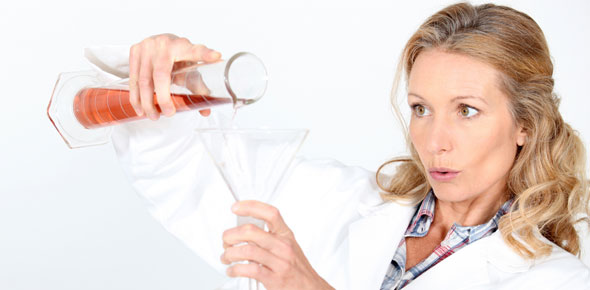# How To Write Chemical Reactions - Chemistry Test

Approved & Edited by ProProfs Editorial Team
At ProProfs Quizzes, our dedicated in-house team of experts takes pride in their work. With a sharp eye for detail, they meticulously review each quiz. This ensures that every quiz, taken by over 100 million users, meets our standards of accuracy, clarity, and engagement.
| Written by Apowell76
A
Apowell76
Community Contributor
Quizzes Created: 1 | Total Attempts: 3,062
Questions: 10 | Attempts: 3,062SettingsA chemical formula is a way of presenting information about the chemical proportions of atoms that constitute a particular chemical compound or molecule, using chemical element symbols, numbers, and sometimes also other symbols, such as parentheses, dashes, brackets, commas and plus and minus signs
Write the chemical formula for each word equation. Be sure to label the correct state of matter for each element/compound.

• 1.

### Solid sodium combines with solution of magnesium fluoride to form sodium fluoride solution and magnesium metal.

Explanation
In this chemical reaction, solid sodium (Na) reacts with a solution of magnesium fluoride (MgF2) to produce sodium fluoride solution (NaF) and solid magnesium (Mg). The reaction can be represented by the balanced chemical equation Na (s) + MgF2 (aq) ----> NaF (aq) + Mg (s).

Rate this question:

• 2.

### Magnesium metal is added to hydrochloric acid to form magnesium chloride solution and hydrogen gas.

Explanation
When magnesium metal is added to hydrochloric acid, a chemical reaction occurs. The magnesium atoms react with the hydrogen ions in the hydrochloric acid to form magnesium chloride, which is soluble in water, and hydrogen gas. This reaction is represented by the balanced chemical equation: Mg (s) + HCl (aq) → MgCl2 (aq) + H2 (g). In this reaction, the magnesium metal loses electrons and is oxidized, while the hydrogen ions gain electrons and are reduced. The formation of magnesium chloride and hydrogen gas is a result of the transfer of electrons between the magnesium and the hydrogen ions.

Rate this question:

• 3.

### Chlorine gas is added to solution of potassium iodide to form potassium chloride solution and iodine liquid.

Explanation
Chlorine gas (Cl2) is added to a solution of potassium iodide (KI) to form potassium chloride (KCl) solution and iodine (I2) liquid. This reaction occurs because chlorine is more reactive than iodine and displaces it from the potassium iodide solution. The chlorine atoms combine with the potassium ions to form potassium chloride, while the iodine is released as a liquid.

Rate this question:

• 4.

### Sodium chloride crystals are heated to form sodium powder and chlorine gas.

Explanation
When sodium chloride crystals are heated, they undergo a chemical reaction and decompose into sodium powder and chlorine gas. This reaction can be represented by the equation NaCl (s) ---> Na (s) + Cl2 (g). The solid sodium chloride breaks down into solid sodium and gaseous chlorine molecules. This reaction occurs due to the high temperature, which provides the energy necessary to break the bonds between the sodium and chlorine atoms in the crystal lattice structure of sodium chloride.

Rate this question:

• 5.

### Sodium crystals are combined with oxygen gas to form sodium oxide solution

Explanation
In this chemical equation, solid sodium (Na) is combined with gaseous oxygen (O2) to form an aqueous solution of sodium oxide (Na2O). The arrow represents the reaction, with the reactants on the left side and the product on the right side. This equation shows the balanced combination of sodium and oxygen atoms to form sodium oxide molecules in solution.

Rate this question:

• 6.

### Sodium crystals are combined with hydrochloric acid to for hydrogen gas and sodium chloride solution.

Explanation
When sodium crystals (Na) are combined with hydrochloric acid (HCl), a chemical reaction occurs. The sodium reacts with the hydrochloric acid to produce hydrogen gas (H2) and sodium chloride solution (NaCl). This reaction can be represented by the balanced chemical equation: Na (s) + HCl (aq) → H2(g) + NaCl(aq).

Rate this question:

• 7.

### Potassium metal is combined with chlorine gas to form potassium chloride solution.

Explanation
In this reaction, solid potassium (K) reacts with gaseous chlorine (Cl2) to form an aqueous solution of potassium chloride (KCl). The arrow indicates the direction of the reaction, with the reactants on the left side and the product on the right side. The state symbols (s, g, aq) indicate the physical state of each substance: solid, gas, or aqueous solution.

Rate this question:

• 8.

### A solution of copper (II) oxide is combined with solid carbon to form copper metal and carbon dioxide gas.

Explanation
In this reaction, copper (II) oxide (CuO) in solution reacts with solid carbon (C) to produce copper metal (Cu) and carbon dioxide gas (CO2). This is a redox reaction where copper (II) oxide is reduced to copper metal, while carbon is oxidized to carbon dioxide. The balanced chemical equation represents the correct stoichiometry of the reaction, with one mole of CuO reacting with one mole of C to produce one mole of Cu and one mole of CO2.

Rate this question:

• 9.

### Calcium metal is combined with oxygen gas to form solid calcium oxide.

Explanation
When calcium metal is combined with oxygen gas, a chemical reaction occurs and solid calcium oxide is formed. This reaction can be represented by the balanced chemical equation: Ca(s) + O2(g) ----> CaO(s). In this reaction, calcium atoms react with oxygen molecules to produce calcium oxide. The solid calcium oxide is formed as a result of the combination of calcium and oxygen atoms.

Rate this question:

• 10.

### Nitrogen gas combines with hydrogen gas to form an ammonia solution.

Related TopicsBack to top
×

Wait!
Here's an interesting quiz for you.